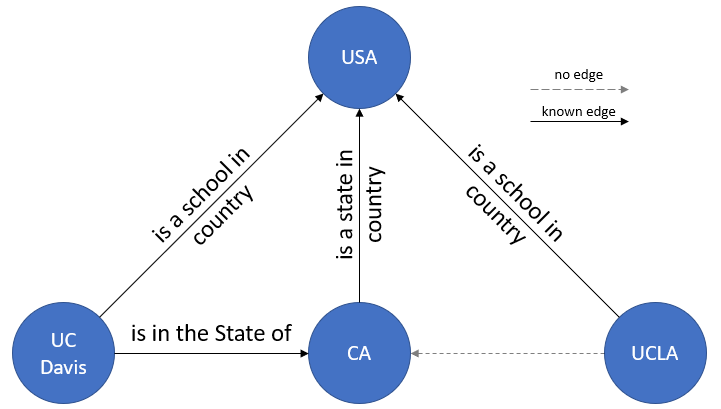Short answer: Knowledge graph completion is the act of inferring new edges, called facts, in a knowledge graph based on the already existing relational data.

Understanding this will require explanation of two concepts, notably 1) what knowledge graph is, and 2) what knowledge graph completion is.  For clarity, use of the term “knowledge graph” here is not meant as Google’s Knowledge Graph , but instead as a knowledge base.

1. So what is a knowledge graph (KG)?

Although you may have previously never heard of knowledge graphs, there’s a chance you may use them multiple times everyday without knowing. There are many applications of KGs, including, but not limited to query expansion , information retrieval, and question answering (like Amazon’s Alexa). Because of its importance in modern digital age, a great number of large-scale KGs such as NELL , Freebase , YAGO2 , and Google’s Knowledge Graph have been recently created. You can simply think of these as massive datasets that share a similar structure.

The structure of a KG is simple – in Figure 1, we have an edge (a.k.a. relation) connecting two nodes (a.k.a. entities). We can also represent this building block in the form of binary relationship, in particular, (subject, predicate, object) triples, where subject and object are the two nodes, and predicate is the edge connecting the two nodes.

Let us assume that we have world’s simplest KG as in Figure 2 where there is only one triple. In this case, our subject is ‘UC Davis’, our predicate is ‘is in the State of’, and our object is ‘CA’. This simple example should give you a better understanding of the basic building components of a KG, and how to visualize it.

2. What is a knowledge graph completion?

Now that we have established a visual intuition behind a KG, let us build a slightly more complicated (but still not so useful) KG.Figure 3. Sample KG where there exists a missing edge between the two nodes ‘UCLA’ and ‘CA’.

In Figure 3, we now have 4 unique nodes and 3 unique edges (note that there are two ‘is a school in country’ edges). In this figure, the solid line denotes that an edge is known, and the dotted line denotes that there is no edge connecting between the two nodes. Now, take a look at the two nodes ‘UCLA’ and ‘CA’. You’ll see that there is no edge connecting the two.

Given this KG, if we want to answer the question “Is UCLA in California?”, how do we do so? We begin by analyzing the relationship between the three nodes (UC Davis, CA, USA). We can see that indeed there is a relation between both the UC Davis and CA nodes and the CA and USA nodes, so we can verify that the statement “UC Davis is in California” to be true. Observing the same structure between the UCLA and CA nodes that we saw in the UC Davis and CA nodes, we can deduce with certain confidence that statement “UCLA is in California” is true as well. Performing this deduction to infer a new edge (UCLA’s relation to CA) in the above KG based on the already existing relational data (UC Davis’s relation to CA’s) is the act of ‘knowledge graph completion’.

So now what?

Knowledge graphs these days are extremely large. Take Google’s Knowledge Graph for example – there are 570 million nodes (entities) and 18,000 million edges (relations), however, there are many many edges that are missing in Google’s Knowledge Graph. The ultimate goal of knowledge graph completion is to be able to fill up all these missing edges. But how do we do it? Stay tuned to my next blog to find out!

References:

1. A. Singhal, “Introducing the Knowledge Graph: things, not strings,” May 2012. [Online]. Available: http://googleblog.blogspot.com/2012/05/ introducing-knowledge-graph-things-not.html
2. J. Graupmann, et al. “The SphereSearch engine for unified ranked retrieval of heterogeneous XML and web documents.”, VLDB, 2005.
3. A. Carlson, et al. “Toward an architecture for never-ending language learning.”, AAAI, 2010.
4. K. Bollacker, et al. “Freebase: a collaboratively created graph database for structuring human knowledge.”, ACM, 2008.
5. J. Hoffart, et al. “YAGO2: A Spatially and Temporally Enhanced Knowledge Base from Wikipedia.”, AIJ, 2012.